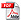A quantum Rosetta stone for interferometry

Hwang Lee, Pieter Kok and Jonathan P. Dowling

Journal of Modern Optics 49, 2325-2338 (2002)Heisenberg-limited measurement protocols can be used to gain an increase in measurement precision over classical protocols. Such measurements can be implemented using, e.g., optical Mach-Zehnder interferometers and Ramsey spectroscopes. We address the formal equivalence between the Mach-Zehnder interferometer, the Ramsey spectroscope, and the discrete Fourier transform. Based on this equivalence we introduce the quantum ``Rosetta stone'', and we describe a projective-measurement scheme for generating the desired correlations between the interferometric input states in order to achieve Heisenberg-limited sensitivity. The Rosetta stone then tells us the same method should work in atom spectroscopy.##### Teaching

© 2019 Pieter Kok